# Cutting Length of Stirrups in Column or Beam | Cutting Length Formula for Reinforcement | Stirrups Length Calculation

In this Article today we will talk about the Cutting Length of Stirrups in Column or Beam | Cutting Length Formula for Reinforcement | Stirrups Length Calculation | Hook Length Formula for Stirrups | Number of Stirrups in Column | BBS of Stirrups

## What is Stirrup:

A stirrup is basically a shear reinforcement wrapped around the longitudinal bars in columns and beams. Cutting Length Formula for Reinforcement

## Purpose of Stirrups in Reinforcement:

In order to achieve the required stress resistance, we have to place required number of bars in respective positions. We have already discussed the load acting on a column in types of column failure where we have explained about the load actions such as compressive and buckling stress. Each load acting on a column will raise some sort of displacement, cracks, and tension in the reinforcement. So the main purpose of stirrups are

• To keep the reinforcement in place
• To resist the flexural shear stress developed in the reinforcement.
• To resist the diagonal tension crack propagation though it is limited

## Method for Calculating Cutting Length of Stirrup:

1. Look at the size of column or beam from drawings
2. Adopt Dia of the bar (generally 8mm Dia is used for stirrups)
3. Deduct the concrete cover or clear cover
4. Find the total outer length of stirrup after deducting concrete cover.
5. Add the length of the hook to the length of the stirrup
6. Deduct the length of bends. Stirrups Length Calculation
7. Use below formula to find the total cutting length of stirrups

Formula:  Cutting Length of Stirrups = Perimeter of Shape + Total hook length – Total Bend Length

### Important Basic Stirrups Length Calculation formulas:

• Perimeter of Rectangle = 2 ( length + breadth)
• Perimeter of Square = 4 x side length
• Perimeter of circle or Circumference of Circle = 2πr = πd (r= radius, d= Diameter of Circle) Number of Stirrups in Column

## Typical Diagram of Stirrup:

Refer the below image of the typical diagram of stirrup for clear understanding about x & y length, bends, hooks and concrete cover.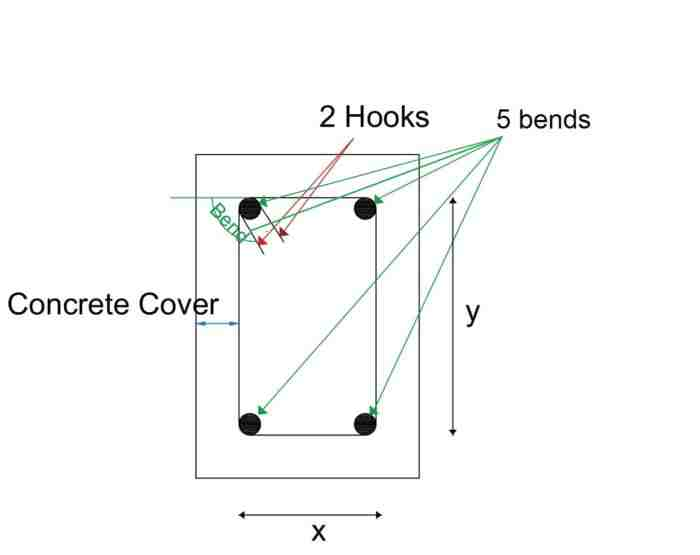In the above image, there are 5 bends at 4 corners, 2 hooks and concrete cover around the stirrup.

x = length of the stirrup in the x-direction after deducting concrete cover &

y = length of the stirrup in the y-direction after deducting concrete cover.

## Important standards used in Bends & Hooks:

The below standards are most important in calculating the hook length and bend lengths at corners while finding cutting length of stirrups.

• 1 Hook length = 9d or 75mm
• 45° Bend length = 1d
• 90° Bend length = 2d
• 135° Bend length = 3d

Remember, d = Diameter of Bar

## Cutting length for Rectangular Stirrups:

Rectangular column or rectangular beam is the most commonly used shape of the column in any construction. In this shape of beam or column, a rectangular stirrup is usually adopted. Number of Stirrups in Column

1. Considering the below Rectangular column size 230mm x 450mm for calculation purpose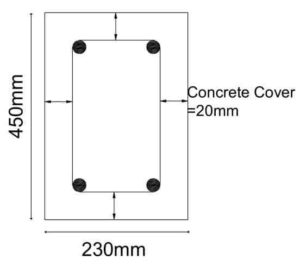2. Adopting Dia of Bar used for stirrups is 8mm
3. Deducting the concrete cover 20mm from all sides                                                        x = 230 – 20-20 = 190mm                                                                                        y = 450-20-20 = 410mm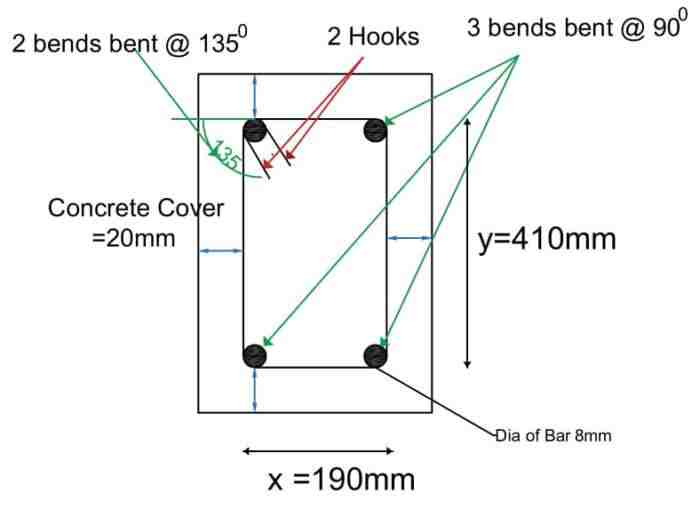4. Total Length of the hooks: From fig, There are two hooks which means 9d+9d = 18d
5. Total Length of Bends: From above fig, There are 3 bends which are bent at an angle of 900 and two bends are bent at an angle of 1350

Total bend length = 3 x 900 Bend length + 2 x 1350 Bend length = 3 x 2d + 2 x 3d = 12d = 12 x 8 = 96mm

Total Cutting length of Rectangular Stirrup = Perimeter of Rectangle + Total Hook length – Total Bend Length

= 2 (x+y) +18d – 12d = 2(190 + 410) + 18 x 8 – 12 x 8 = 1248mm = 1.248m

## Cutting length for Square Stirrups:

1. Considered the column size as 450mm x 450mm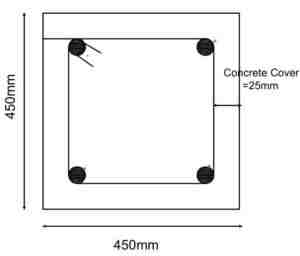2. Adopting Dia of Bar used for stirrups is 8mm
3. Deducting the concrete cover 25mm from all sides (in square all sides are equal)            x = 450- 20-20 = 410mm                                                                                          y = 450-20-20 = 410mm, Hence x = y (in square all sides are equal).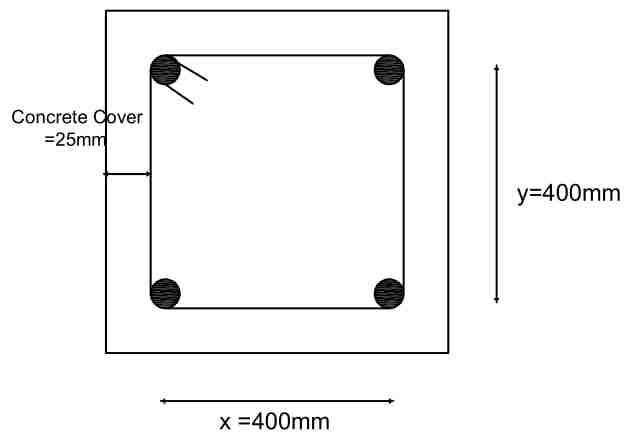4. Total Length of the hook: There are two hooks which mean 9d+9d = 18d
5. Total length of Bends: There are 3 bends which are bent at an angle of 900 and one is bent at an angle of 1350.                                                                                          Total bend length = 3 x 900                                                                                    Bend length + 2 x 1350                                                                                          Bend length = 3 x 2d + 2 x 3d = 12d = 12 x 8 = 96mm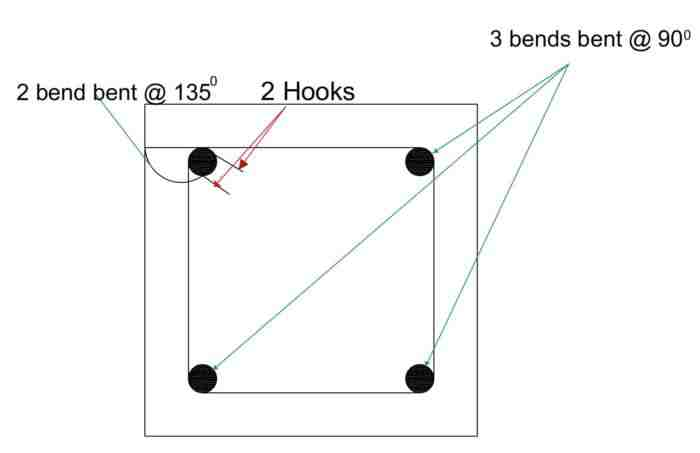Total Cutting length of Square Stirrup = Perimeter of Square + Total Hook length – Total Bend Length

= 4 x 410 +18d – 12d = 1648mm = 1.64m

## Cutting Length for Circular Stirrup:

1. Considered the column dia as D = 1000mm
2. Adopting Dia of Bar used for stirrups is d =8mm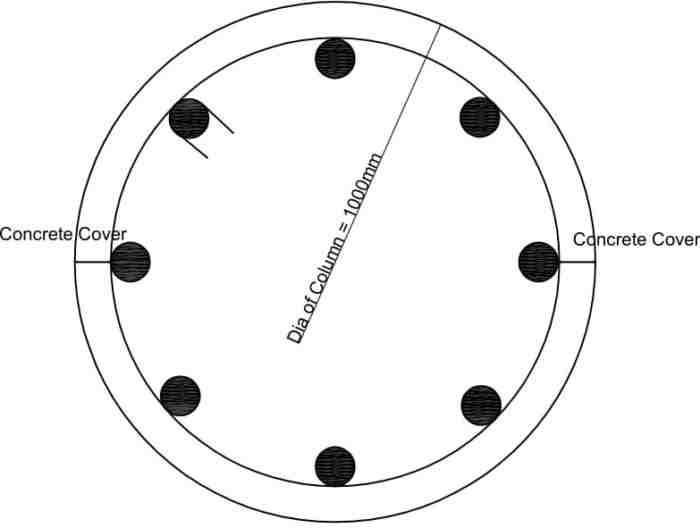3. Deducting the concrete cover from diameter of column D = 1000-25-25 = 950mm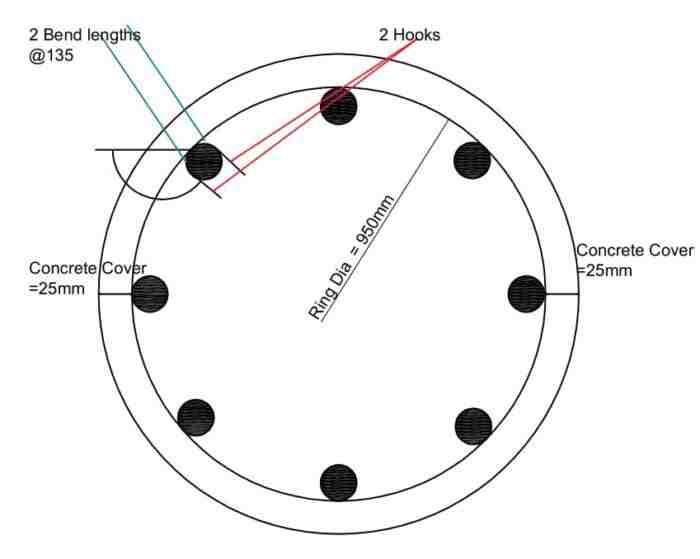4. Circumference length of Ring = πD = 950 x 3.14 = 2983mm
5. Total Length of the hook: There are two hooks which means 9d+9d= 18d
6. Total Length of Bends: There are 2 bends which are bent at an angle of 1350 Total bend length = 2 x 1350                                                                                          Bend length = 2 x 3d = 6d= 6 x 8 = 48mm

Total Cutting length of Circular Stirrup or Ring = Circumference of Circle + Total Hook length – Total Bend Length

= 2983 +18d – 6d =3079mm =3.07m

## Cutting Length for Triangular Stirrups:

1. Considered the Column size 400mm x 450mm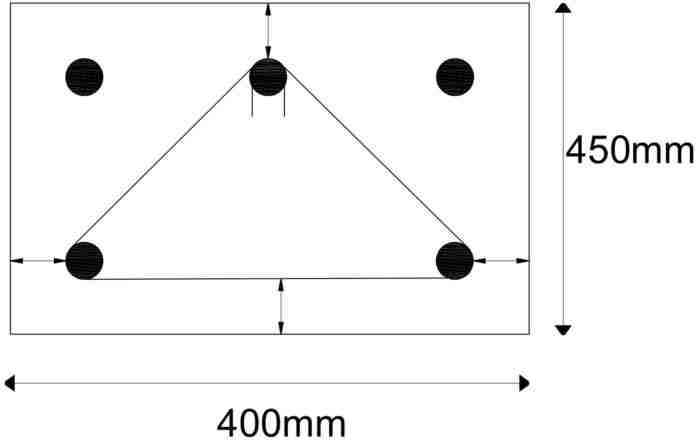2. Adopting Dia of Bar used for stirrups is d = 8mm
3. Deducting the concrete cover 25mm from all sides                                                        x = 400-20-20 = 360mm                                                                                            y = 450-20-20 = 410mm                                                                                          From Pythagorean theorem,                                                                          Hypotenuse2=(Opposite)2 + (Adjacent)2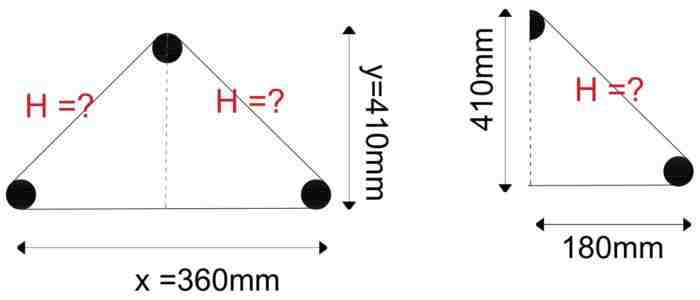look at 2nd triangle in above image H2=(x/2)2 + y2                                                            H2=1802 + 4102 => =  √(447)2  = 447mm                                                                The total length of stirrup till now = 2 x H + 360 = 2 x 447 + 360 = 1254mm
4. Total Length of the hooks:                                                                                       There are two hooks which means 9d+9d= 18d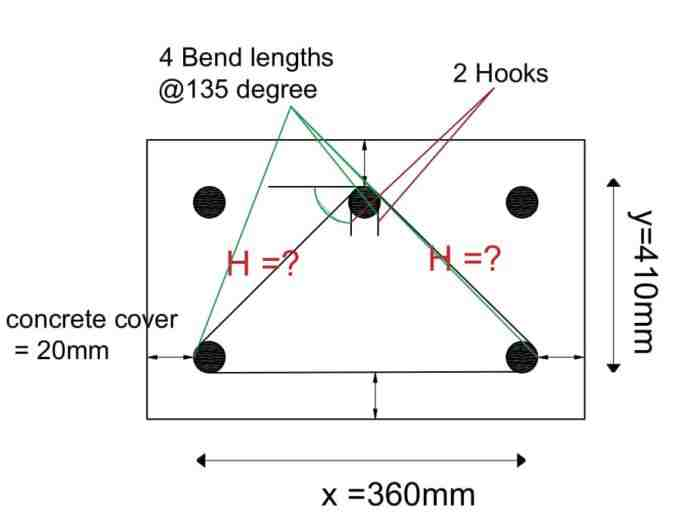5. Total length of Bends:                                                                                        There are 4 bends which are bent at an angle of 1350                                                Total bend length =4 x 1350 Bend length=  4 x 3d = 12d= 12 x 8 = 96mm

Total Cutting length of Triangular Stirrup = Perimeter of Triangle + Total Hook length – Total Bend Length

= 1254+18d – 12d = 1302mm = 1.3m

## Cutting Length for Diamond Stirrups:

1. Considered the Column size 400mm x 400mm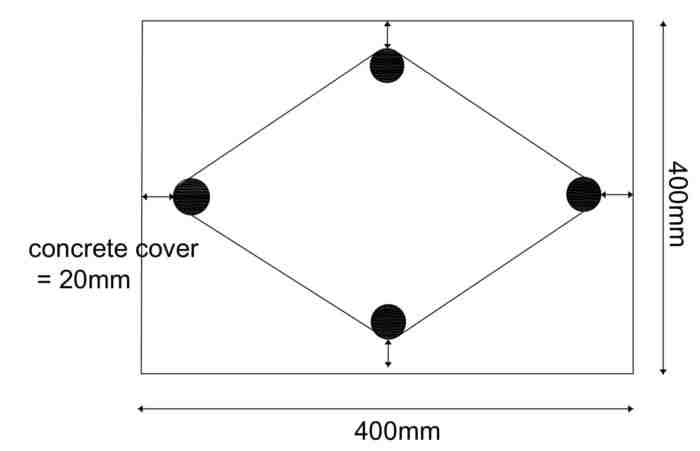2. Adopting Dia of Bar used for stirrups is d = 8mm. Hook Length Formula for Stirrups
3. Deducting the concrete cover 25mm from all sides                                                      x = 400-20-20 = 360mm                                                                                            y = 400-20-20 =360mm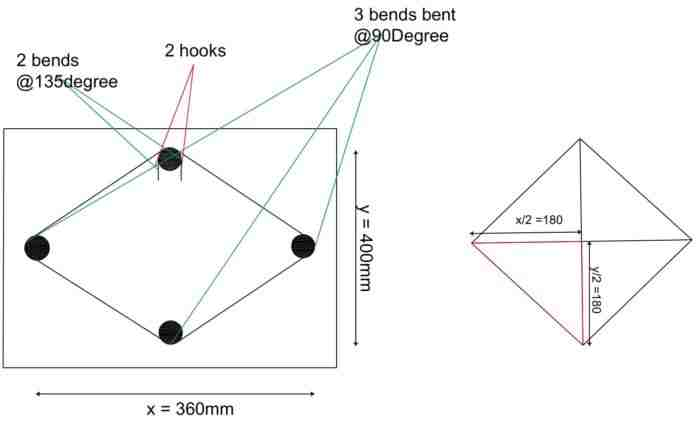From Pythagorean theorem,                                                                                    Hypotenuse2=(Opposite)2 + (Adjacent)2                                                             H2=(x/2)2 +( y/2)2 H2=1802 + 1802 => =  √(254)2  = 254mm
4. The total length of stirrup = 4 x H  =4 x 254 = 1016mm
5. Total Length of the hook: There are two hooks which means 9d+9d= 18d
6. Total length of Bends: There are 3 bends which are bent at an angle of 90 + 2 bends which are bent at an angle of 1350                                                                          Total bend length = 3 x 900 Bend length + 2 x 1350 Bend length= 3 x 2d + 2 x 3d = 12d = 12 x 8 = 96mm

Total Cutting length of Diamond  Stirrup = Perimeter of Diamond shape + Total Hook length – Total Bend Length

= 1016+144-96 = 1064mm = 1.064mm

## Cutting Length for Helical Stirrups: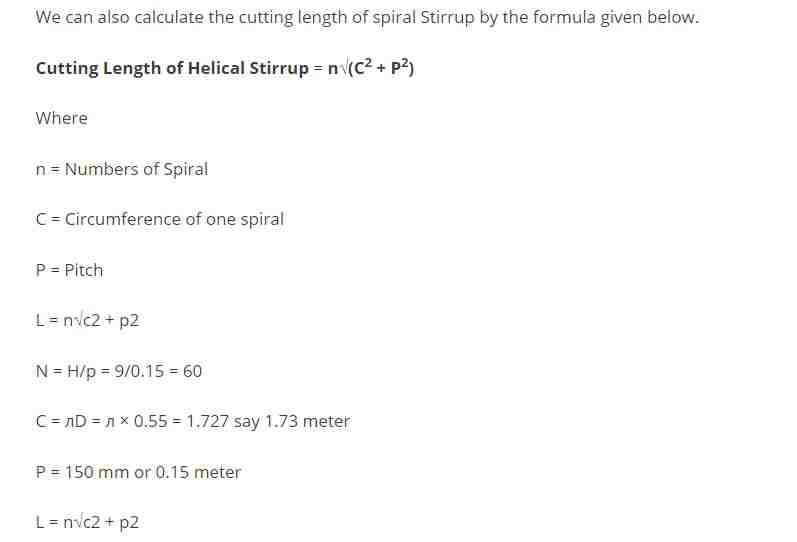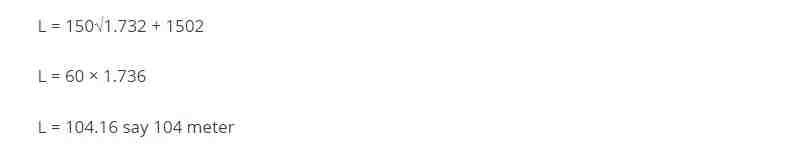I hope now you have enough knowledge on how to calculate the cutting length of stirrups for different shapes. Number of Stirrups in Column

## Conclusion:

Full article on Cutting Length of Stirrups in Column or Beam | Cutting Length Formula for Reinforcement | Stirrups Length Calculation | Hook Length Formula for Stirrups | Number of Stirrups in Column | BBS of Stirrups. Thank you for the full reading of this article in “The Civil Engineering” platform in English. If you find this post helpful, then help others by sharing it on social media. If any formula of BBS is missing from this article please tell me in comments.

1.Satish Kumar Mishra

It’s good

• Thanks for the comments please visit more articles by clicking on “All Posts” tab of the Menu Bar

2.Fasiku

Quite educative ,well done
But why the provision for hook length not for all the vertical rebar ?

3.kiran